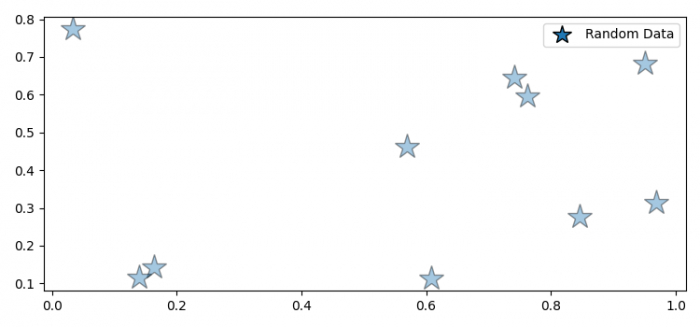# How to set legend marker size and alpha in Matplotlib?

To set legend marker size and alpha in matplotlib, we can take the following steps −

## Steps

• Set the figure size and adjust the padding between and around the subplots.

• Initialize a variable N to store the number of sample data.

• Plot the x and y data points with marker="*".

• Place a legend on the figure.

• Set the marker size and alpha value of the marker.

• To display the figure, use show() method.

## Example

import matplotlib.pyplot as plt
import numpy as np

plt.rcParams["figure.figsize"] = [7.50, 3.50]
plt.rcParams["figure.autolayout"] = True

N = 10

x = np.random.rand(N)
y = np.random.rand(N)

line, = plt.plot(x, y, marker='*', markersize=20, markeredgecolor='black',
alpha=0.4, ls='none', label='Random Data')

legend = plt.legend(loc='upper right')
legend.legendHandles._legmarker.set_markersize(15)
legend.legendHandles._legmarker.set_alpha(1)

plt.show()

## Output

It will produce the following output −